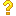•闪存
•博客
• 发言小组
• 投递新闻
• 提问博问
• 添加收藏
• 发布招聘
•文库

0

##下面的 JS 代码是什么意思

<script>[][([]+[])[+[]]+([[]]+[][[]])[+!+[]+[+[]]]+([]+[])[!+[]+!+[]]+(![]+[])[+[]]+(![]+[])[!+[]+!+
3

2

0

2

2

1